Function Repository Resource:

OverfullPieChart

Make a pie chart that exceeds the allowed total

Contributed by: Sander Huisman
 ResourceFunction["OverfullPieChart"][{y1,y2,y3,…},max] make a 3D pie chart of the data yi for which the maximum is max, bending it in 3D to make extra space. ResourceFunction["OverfullPieChart"][{y1,y2,y3,…},max,n] use a mode n bending of the surface.

Details

For pie charts sometimes a little extra space is needed when the values added up exceed the maximum allowed. (i.e. over 100%). When more than 100% is needed, the paper sheet can be bent to create some extra space in the 3rd dimension.

Examples

Basic Examples (1)

Make a pie chart for which the total is 140 but the allowed total is only 100:

 In:=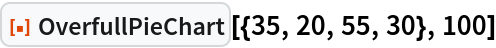Out=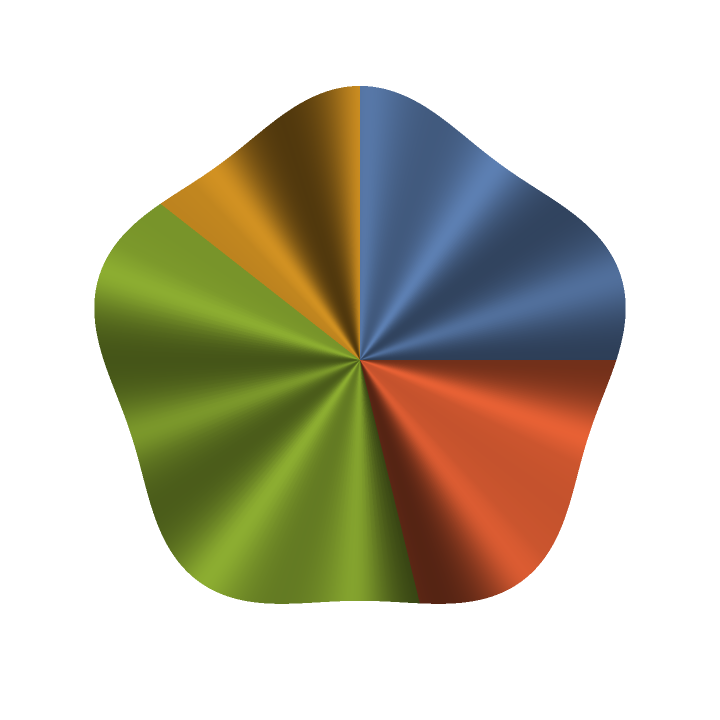Scope (2)

Without a maximum given, the maximum is assumed to be 1:

 In:=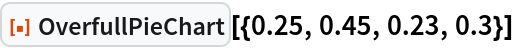Out=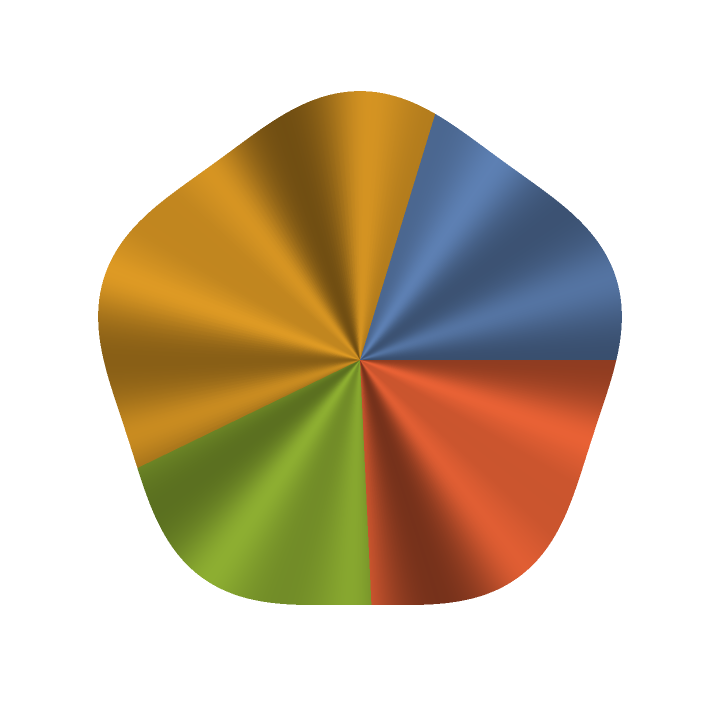Use a higher mode of bending the paper:

 In:=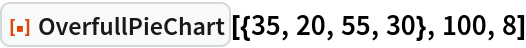Out=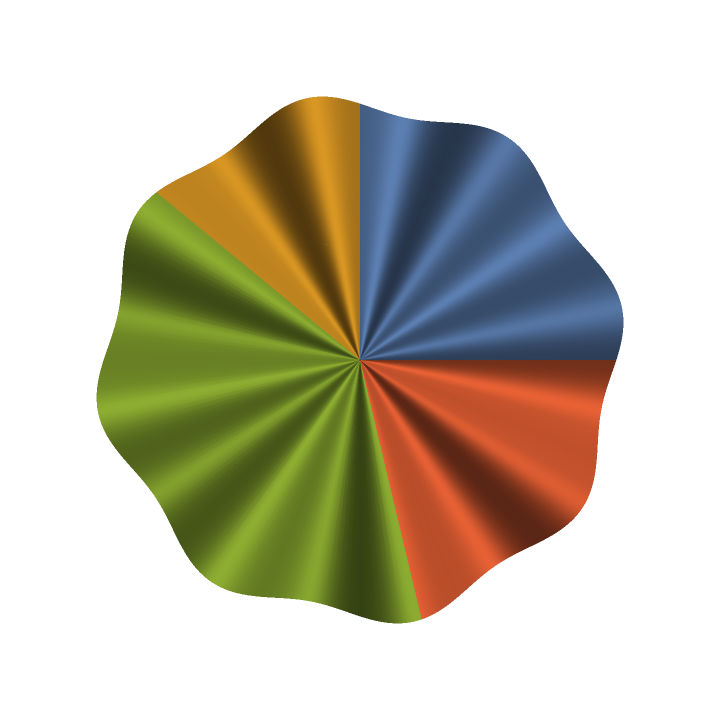Properties and Relations (1)

Compare a regular pie chart and an overfull pie chart:

 In:=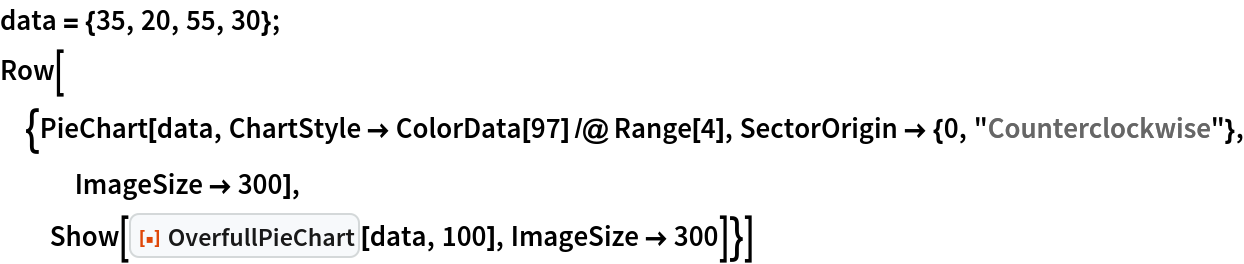Out=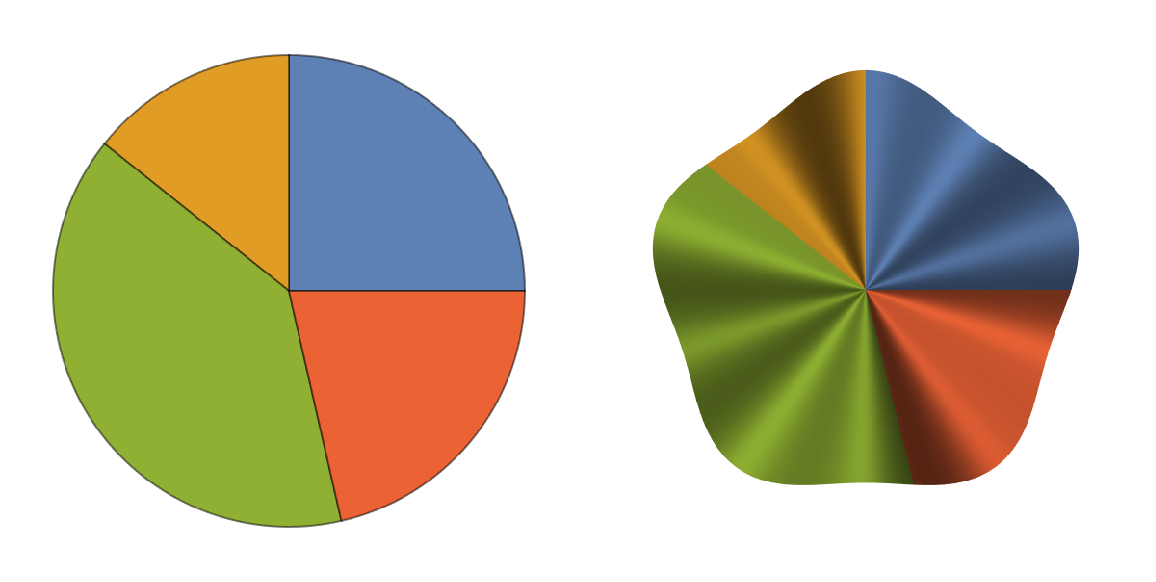Possible Issues (1)

If the total is less than the maximum, the paper can not bend without ripping it:

 In:=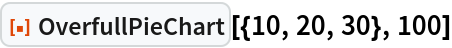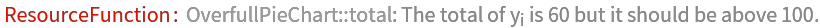Neat Examples (2)

See how it bends the sheet in 3D:

 In:=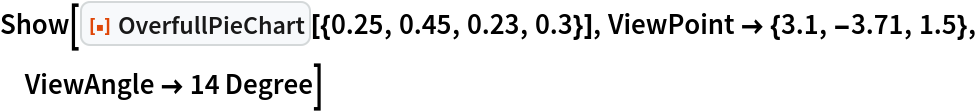Out=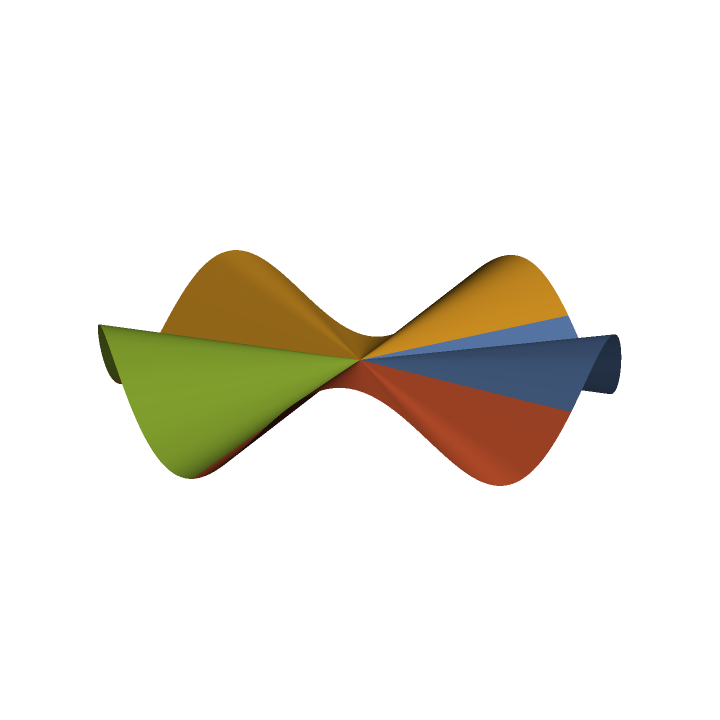For higher modes the amplitude is obviously smaller:

 In:=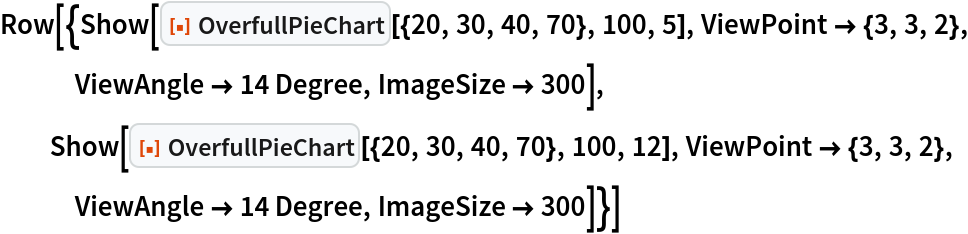Out=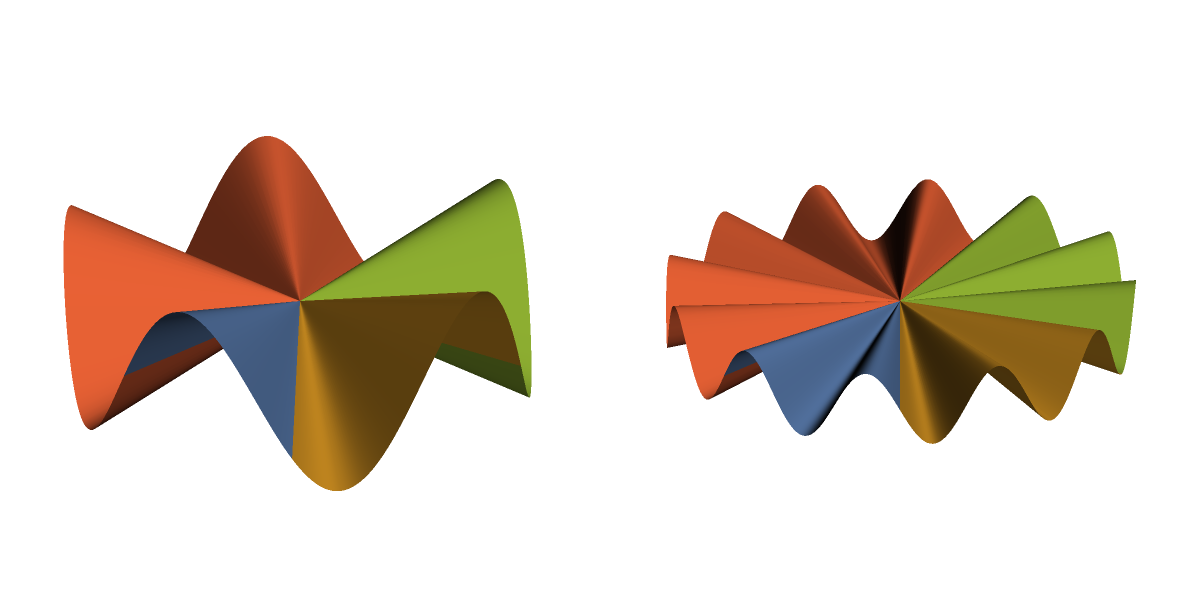Author Notes

Inspired by XKCD comic 2031, where 130% of 'paper' is folded in 3D to make more space.